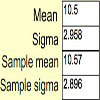#### You may also like### Spinners

How do scores on dice and factors of polynomials relate to each other?### Data Matching

Use your skill and judgement to match the sets of random data.### Random Inequalities

Can you build a distribution with the maximum theoretical spread?

# Time to Evolve 2

##### Age 16 to 18Challenge Level
Suppose that a certain species of mammal matures at the age of 5 and can live to the age of 15. Between maturity and death, the animal can produce offspring.

What is the longest span of time possible between the birth of an animal and the birth of one of its grandparents? What is the shortest possible such time?

It is suggested that the distribution $T_2$ of the time between the birth of an individual and the birth of its parent could be modelled by
$$T_2 = 5 + 10 \mbox{U}[0,1]\,,$$
where $\mbox{U}[0,1]$ is the standard uniform distribution. Do you think that this is a good modelling assumption? What are its strengths and weaknesses? Sketch the pdf of $T$ and overlay this with a sketch of a pdf which you feel would more accurately model the time $T$.

Repeat this analysis for $T_3$, representing child-parent-grandparent. How might you sketch the the pdf of $T_3$? What problems would arise with drawing it accurately, and what parts could you plot exactly?

A chain linking the births of 10 of these animals will have some length $T_{10}$. How would you model the distribution of $T_{10}$ if you used a uniform assumption of the time between birth of offspring and parents as in the previous part of the question? What would be the expectations and standard deviations of $T_{2}, T_3$ and $T_{10}$?

Extension work using numerical simulation
Use a spreadsheet to run 1000 numerical trials of a time period $T_3$. Once you are sure that your sheet works, extend this to make an experimental plot of the pdf of $T_{10}$. Do your results seems to make sense? What do you think the theoretical pdf might look like? What do you think the pdf of $T_{100}$ would look like?

NOTES AND BACKGROUND
The distribution of a sum of independent uniform distributions can be worked out exactly, but involves advanced university level mathematics. You can read more about this idea on the Wolfram MathWorld site.